|

# 金螳螂•家 “520 为你而来”VR发布会隆重开幕

（2017年5月20日 苏州讯）在家装行业，“整装”概念一直被提及，在过去的两年内，以整装模式为代表的互联网品牌获得了飞速的成长，然而并没有实现真正的一体化整装。自金螳螂家在周年庆上提出成为以家庭消费为核心的综合服务商时，金螳螂家就从未停止前进的步伐。

5月20日，金螳螂家新开苏州新区店、宜宾店、泰兴店、胶州店、马鞍山店、连云港店、衢州开化店、常州武进店、泸州店、包头店、南京江宁店、呼和浩特店、无锡滨湖店、苏州吴中店、柳州店、南宁店、义乌店、赣州店、沈阳店、运城店20家门店，至此，金螳螂家已在全国开设101家门店。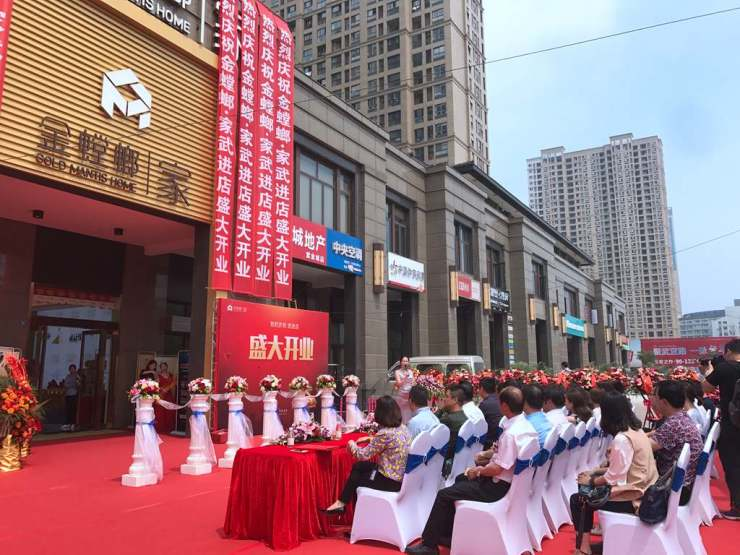借“百城百店”之际，金螳螂家将发布一场别具生面的VR发布会—— “520 为你而来----百城百店放心品质”。消费者通过金螳螂家门店提供的VR眼镜即可观看到整场发布会的盛况。

致敬“品质”金螳螂家战略纵向深发展

近几年，“整装”概念席卷家装行业。整装，通常指为消费者提供完整的装修整体解决方案，它不仅包括以吊顶、墙面、主材为主的硬装解决方案，还包括家具、灯具、窗帘等软装解决方案，更包含品质生活所必须的家居智能系统、健康系统等解决方案。因此，整装的概念已经超出了传统的装修范畴，更注重生活场景、健康生活方式的设计。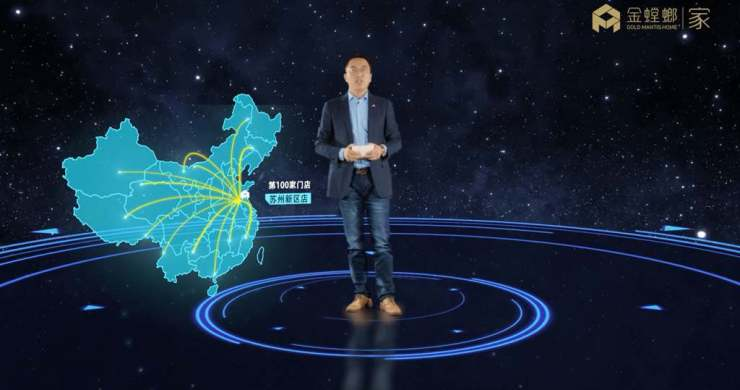为满足消费者多样化、整体的家装需求，金螳螂家秉承着“创造美好生活空间”的品牌使命，推出一体化整装解决方案。同时，基于“标准化中体现个性化、个性化中融合标准化”的原则，金螳螂家不断创新产品、不断整合各类资源，构建整装服务生态系统，为广大客户提供家庭装修（硬装）服务的同时，围绕用户家庭生活各类需求将提供家居装饰（软装）、家居智能、家居健康、家居生活等各类服务。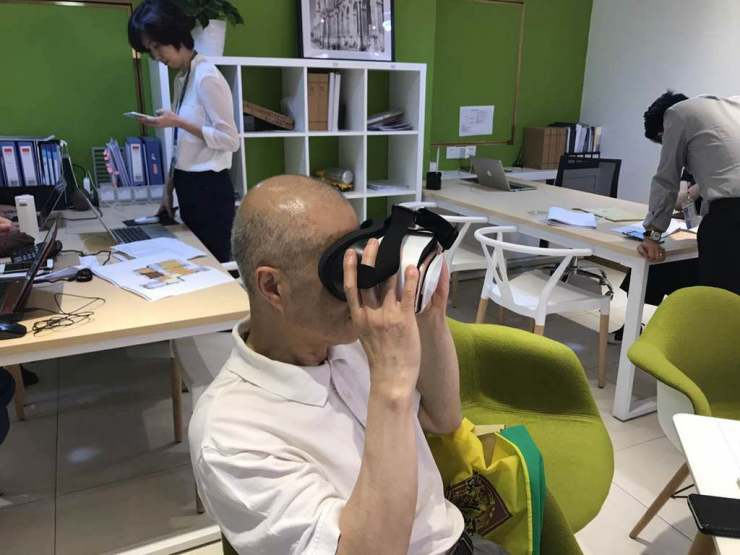5月20日，金螳螂家以“品质生活”为中心，以满足消费者需求未己任，正式推出家居装饰（软装）、家居智能、家居健康系列产品。

金螳螂电商董事长杨鹏说，金螳螂家围绕用户家庭生活全产业链，为消费者打造“健康的一站式整装生活圈”。在这个生态圈中，消费者可以体验到更加畅快、便利的家装体验，VR虚拟现实，所见即所得；24年施工管理经验的匠心独运；4000多名设计师海量案例库的共享……一体化整装服务解决方案的推出，不仅将加快金螳螂家全面进军全国市场的步伐，也将助力金螳螂家纵向领域的发展。金螳螂家用心、专心、且有信心做好每一户装修，精心打造每一个爱家。

值得注意的是，金螳螂家不仅在发布会的形式上做出吸引互联网家装甚或年轻一代的消费者，更为一体化整装服务打出了极具诚意的产品，5月20日公开其软装、智能、健康产品的套餐价格，让更多消费者享受到整装带来的优惠便利。

“放心”家装金螳螂家解读整装服务

“一体化整装服务解决方案”是金螳螂家解读整装后推出的服务。整装不是用简单粗暴的产品轰开市场即可，而是通过迭代好产品同时，对供应链、施工、交付、工期、服务等整个功底不断打造，而金螳螂家软装、智能、健康系列产品就是很好的证明。

硬装只是开始，软装无缝对接。金螳螂家软装产品，传承金螳螂的设计底蕴，从风格设计、产品的选择、供应链集采上，都凸显出了独有的优势。在人性化设计方面，金螳螂家遵循人体工学原理，通过对人居生活的模拟，设计出适应消费者生活起居习惯的软装产品。在环保方面，金螳螂家坚持原材料与产地可追溯原则，从源头把控、层层监督，为消费者提供环保、品质的产品。此次，金螳螂家推出的软装产品，有6大主流风格（现代、北欧、田园、简美、新中式、新古典），实行1+N的产品组合，“1”满足基本需求，是对基本空间客厅、餐厅、卧室的设计，共17件产品，“N”为个性空间，比如儿童房、老人房等。自由组合搭配下，消费者可以有34种选择，满足大多数消费者的需求。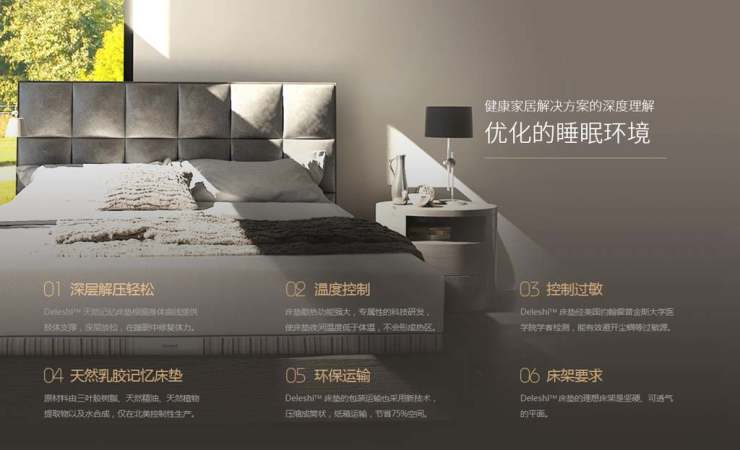家居智能产品包的推出，让金螳螂家在家居智能方面展现出不同的价值。金螳螂家智能产品，是金螳螂家希望从产品设计、价格定位及产品使用等多方面，提升家庭生活的品质，并让每个操作都简单易懂，让智能科技拉近消费者与家的距离，特别是在场景营造、安全防护方面。“情景闹钟”，清晨让灯光渐渐亮起、缓缓拉开窗帘、慢慢提升空调温度，打开新风系统，温柔唤醒熟睡中的人；“厨房安防”，探测厨房中是否有可燃气体泄漏，如遇泄漏可联动手机预警提醒消费者……除此之外，更提供离家场景、远程管控、回家场景、影院场景、阅读场景、睡眠场景等。据说，随便一种场景都能刷新生活方式，大大便利人们的日常。正如金螳螂家所介绍，每一件智能产品，在用材更精致，设计更用心，金螳螂家更想为消费者提供“品质上乘”的服务。

为了让消费者体验智能家居的同时也能享受到健康家居的呵护，金螳螂家为消费者提供了家居健康产品包，金螳螂家家居健康产品包由金螳螂家与美国Delos公司携手完成。Delos与全球著名的梅奥医学研究中心合办健康人居实验室（WELL Living LabTM），通过最先进的感应系统以及模块化的空间设置，模拟不同类型的人居环境，通过真人对环境的实时反应来监测、研究环境对人体健康的影响，并不断将研究成果应用于生活场景中。金螳螂•家By Delos™围绕着深度净化空气、水、动态灯光、睡眠环境四大系统提供健康家居解决方案，在消费者带来一个新的健康生活方式。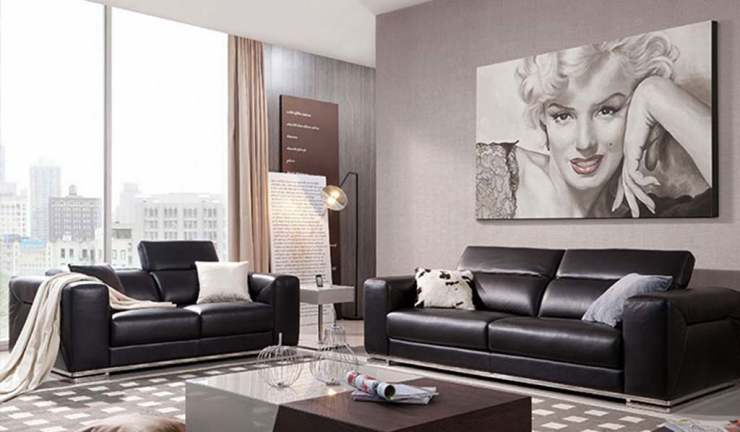金螳螂家，一直致力于为消费者提供家庭装修、家居装饰、家居智能、家居健康、家居生活等以一体化整装服务解决方案，未来，金螳螂家也将奋勇前进，为更多的消费者提供放心、品质的家装服务。

`声明：本文由入驻焦点开放平台的作者撰写，除焦点官方账号外，观点仅代表作者本人，不代表焦点立场错误信息举报电话： 400-099-0099，邮箱：jubao@vip.sohu.com，或点此进行意见反馈，或点此进行举报投诉。`A B C D E F G H J K L M N P Q R S T W X Y Z
A - B - C - D - E
• A
• 鞍山
• 安庆
• 安阳
• 安顺
• 安康
• 澳门
• B
• 北京
• 保定
• 包头
• 巴彦淖尔
• 本溪
• 蚌埠
• 亳州
• 滨州
• 北海
• 百色
• 巴中
• 毕节
• 保山
• 宝鸡
• 白银
• 巴州
• C
• 承德
• 沧州
• 长治
• 赤峰
• 朝阳
• 长春
• 常州
• 滁州
• 池州
• 长沙
• 常德
• 郴州
• 潮州
• 崇左
• 重庆
• 成都
• 楚雄
• 昌都
• 慈溪
• 常熟
• D
• 大同
• 大连
• 丹东
• 大庆
• 东营
• 德州
• 东莞
• 德阳
• 达州
• 大理
• 德宏
• 定西
• 儋州
• 东平
• E
• 鄂尔多斯
• 鄂州
• 恩施
F - G - H - I - J
• F
• 抚顺
• 阜新
• 阜阳
• 福州
• 抚州
• 佛山
• 防城港
• G
• 赣州
• 广州
• 桂林
• 贵港
• 广元
• 广安
• 贵阳
• 固原
• H
• 邯郸
• 衡水
• 呼和浩特
• 呼伦贝尔
• 葫芦岛
• 哈尔滨
• 黑河
• 淮安
• 杭州
• 湖州
• 合肥
• 淮南
• 淮北
• 黄山
• 菏泽
• 鹤壁
• 黄石
• 黄冈
• 衡阳
• 怀化
• 惠州
• 河源
• 贺州
• 河池
• 海口
• 红河
• 汉中
• 海东
• 怀来
• I
• J
• 晋中
• 锦州
• 吉林
• 鸡西
• 佳木斯
• 嘉兴
• 金华
• 景德镇
• 九江
• 吉安
• 济南
• 济宁
• 焦作
• 荆门
• 荆州
• 江门
• 揭阳
• 金昌
• 酒泉
• 嘉峪关
K - L - M - N - P
• K
• 开封
• 昆明
• 昆山
• L
• 廊坊
• 临汾
• 辽阳
• 连云港
• 丽水
• 六安
• 龙岩
• 莱芜
• 临沂
• 聊城
• 洛阳
• 漯河
• 娄底
• 柳州
• 来宾
• 泸州
• 乐山
• 六盘水
• 丽江
• 临沧
• 拉萨
• 林芝
• 兰州
• 陇南
• M
• 牡丹江
• 马鞍山
• 茂名
• 梅州
• 绵阳
• 眉山
• N
• 南京
• 南通
• 宁波
• 南平
• 宁德
• 南昌
• 南阳
• 南宁
• 内江
• 南充
• P
• 盘锦
• 莆田
• 平顶山
• 濮阳
• 攀枝花
• 普洱
• 平凉
Q - R - S - T - W
• Q
• 秦皇岛
• 齐齐哈尔
• 衢州
• 泉州
• 青岛
• 清远
• 钦州
• 黔南
• 曲靖
• 庆阳
• R
• 日照
• 日喀则
• S
• 石家庄
• 沈阳
• 双鸭山
• 绥化
• 上海
• 苏州
• 宿迁
• 绍兴
• 宿州
• 三明
• 上饶
• 三门峡
• 商丘
• 十堰
• 随州
• 邵阳
• 韶关
• 深圳
• 汕头
• 汕尾
• 三亚
• 三沙
• 遂宁
• 山南
• 商洛
• 石嘴山
• T
• 天津
• 唐山
• 太原
• 通辽
• 铁岭
• 泰州
• 台州
• 铜陵
• 泰安
• 铜仁
• 铜川
• 天水
• 天门
• W
• 乌海
• 乌兰察布
• 无锡
• 温州
• 芜湖
• 潍坊
• 威海
• 武汉
• 梧州
• 渭南
• 武威
• 吴忠
• 乌鲁木齐
X - Y - Z
• X
• 邢台
• 徐州
• 宣城
• 厦门
• 新乡
• 许昌
• 信阳
• 襄阳
• 孝感
• 咸宁
• 湘潭
• 湘西
• 西双版纳
• 西安
• 咸阳
• 西宁
• 仙桃
• 西昌
• Y
• 运城
• 营口
• 盐城
• 扬州
• 鹰潭
• 宜春
• 烟台
• 宜昌
• 岳阳
• 益阳
• 永州
• 阳江
• 云浮
• 玉林
• 宜宾
• 雅安
• 玉溪
• 延安
• 榆林
• 银川
• Z
• 张家口
• 镇江
• 舟山
• 漳州
• 淄博
• 枣庄
• 郑州
• 周口
• 驻马店
• 株洲
• 张家界
• 珠海
• 湛江
• 肇庆
• 中山
• 自贡
• 资阳
• 遵义
• 昭通
• 张掖
• 中卫

1室1厅1厨1卫1阳台

1
2
3
4
5

0
1
2

1

1

0
1
2
3报名成功，资料已提交审核A B C D E F G H J K L M N P Q R S T W X Y Z
A - B - C - D - E
• A
• 鞍山
• 安庆
• 安阳
• 安顺
• 安康
• 澳门
• B
• 北京
• 保定
• 包头
• 巴彦淖尔
• 本溪
• 蚌埠
• 亳州
• 滨州
• 北海
• 百色
• 巴中
• 毕节
• 保山
• 宝鸡
• 白银
• 巴州
• C
• 承德
• 沧州
• 长治
• 赤峰
• 朝阳
• 长春
• 常州
• 滁州
• 池州
• 长沙
• 常德
• 郴州
• 潮州
• 崇左
• 重庆
• 成都
• 楚雄
• 昌都
• 慈溪
• 常熟
• D
• 大同
• 大连
• 丹东
• 大庆
• 东营
• 德州
• 东莞
• 德阳
• 达州
• 大理
• 德宏
• 定西
• 儋州
• 东平
• E
• 鄂尔多斯
• 鄂州
• 恩施
F - G - H - I - J
• F
• 抚顺
• 阜新
• 阜阳
• 福州
• 抚州
• 佛山
• 防城港
• G
• 赣州
• 广州
• 桂林
• 贵港
• 广元
• 广安
• 贵阳
• 固原
• H
• 邯郸
• 衡水
• 呼和浩特
• 呼伦贝尔
• 葫芦岛
• 哈尔滨
• 黑河
• 淮安
• 杭州
• 湖州
• 合肥
• 淮南
• 淮北
• 黄山
• 菏泽
• 鹤壁
• 黄石
• 黄冈
• 衡阳
• 怀化
• 惠州
• 河源
• 贺州
• 河池
• 海口
• 红河
• 汉中
• 海东
• 怀来
• I
• J
• 晋中
• 锦州
• 吉林
• 鸡西
• 佳木斯
• 嘉兴
• 金华
• 景德镇
• 九江
• 吉安
• 济南
• 济宁
• 焦作
• 荆门
• 荆州
• 江门
• 揭阳
• 金昌
• 酒泉
• 嘉峪关
K - L - M - N - P
• K
• 开封
• 昆明
• 昆山
• L
• 廊坊
• 临汾
• 辽阳
• 连云港
• 丽水
• 六安
• 龙岩
• 莱芜
• 临沂
• 聊城
• 洛阳
• 漯河
• 娄底
• 柳州
• 来宾
• 泸州
• 乐山
• 六盘水
• 丽江
• 临沧
• 拉萨
• 林芝
• 兰州
• 陇南
• M
• 牡丹江
• 马鞍山
• 茂名
• 梅州
• 绵阳
• 眉山
• N
• 南京
• 南通
• 宁波
• 南平
• 宁德
• 南昌
• 南阳
• 南宁
• 内江
• 南充
• P
• 盘锦
• 莆田
• 平顶山
• 濮阳
• 攀枝花
• 普洱
• 平凉
Q - R - S - T - W
• Q
• 秦皇岛
• 齐齐哈尔
• 衢州
• 泉州
• 青岛
• 清远
• 钦州
• 黔南
• 曲靖
• 庆阳
• R
• 日照
• 日喀则
• S
• 石家庄
• 沈阳
• 双鸭山
• 绥化
• 上海
• 苏州
• 宿迁
• 绍兴
• 宿州
• 三明
• 上饶
• 三门峡
• 商丘
• 十堰
• 随州
• 邵阳
• 韶关
• 深圳
• 汕头
• 汕尾
• 三亚
• 三沙
• 遂宁
• 山南
• 商洛
• 石嘴山
• T
• 天津
• 唐山
• 太原
• 通辽
• 铁岭
• 泰州
• 台州
• 铜陵
• 泰安
• 铜仁
• 铜川
• 天水
• 天门
• W
• 乌海
• 乌兰察布
• 无锡
• 温州
• 芜湖
• 潍坊
• 威海
• 武汉
• 梧州
• 渭南
• 武威
• 吴忠
• 乌鲁木齐
X - Y - Z
• X
• 邢台
• 徐州
• 宣城
• 厦门
• 新乡
• 许昌
• 信阳
• 襄阳
• 孝感
• 咸宁
• 湘潭
• 湘西
• 西双版纳
• 西安
• 咸阳
• 西宁
• 仙桃
• 西昌
• Y
• 运城
• 营口
• 盐城
• 扬州
• 鹰潭
• 宜春
• 烟台
• 宜昌
• 岳阳
• 益阳
• 永州
• 阳江
• 云浮
• 玉林
• 宜宾
• 雅安
• 玉溪
• 延安
• 榆林
• 银川
• Z
• 张家口
• 镇江
• 舟山
• 漳州
• 淄博
• 枣庄
• 郑州
• 周口
• 驻马店
• 株洲
• 张家界
• 珠海
• 湛江
• 肇庆
• 中山
• 自贡
• 资阳
• 遵义
• 昭通
• 张掖
• 中卫• 手机• 分享
• 设计
免费设计
• 计算器
装修计算器
• 入驻
合作入驻
• 联系
联系我们
• 置顶
返回顶部